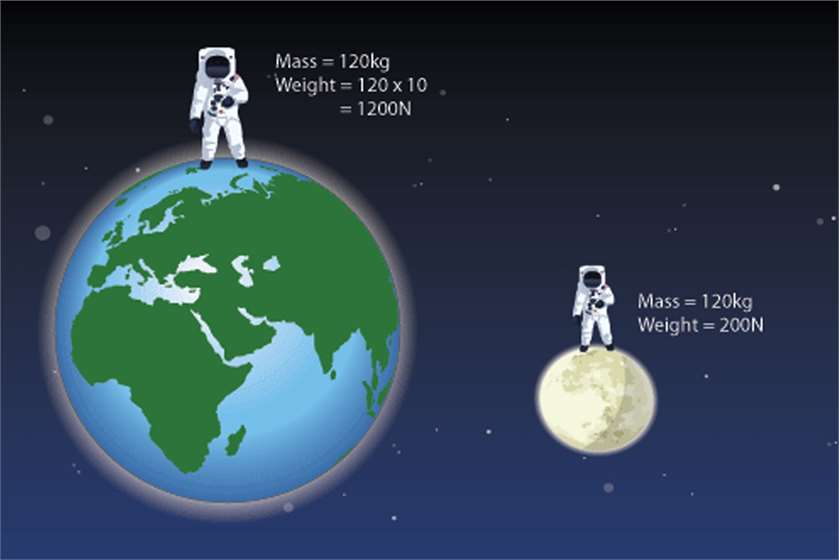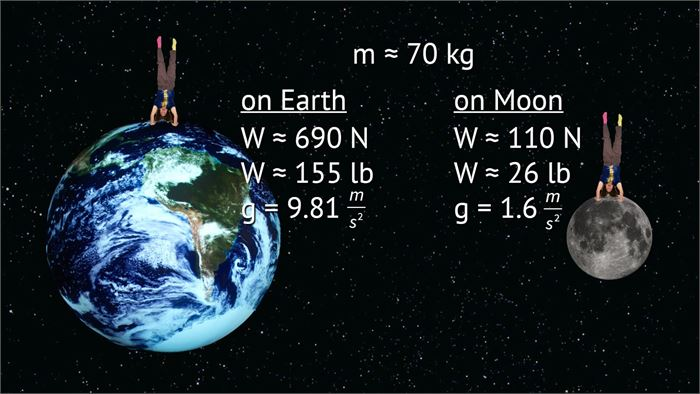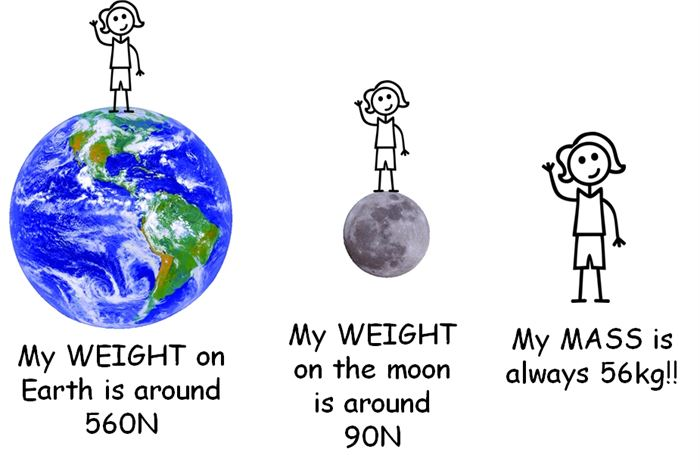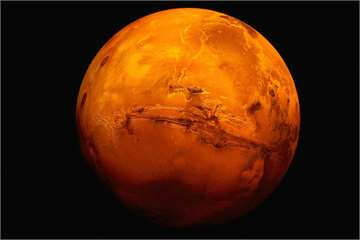# What is the difference between Weight and Mass?

2,878 Views Updated: 10 Sep 2017The team “weight” and “mass” has been used often in conversation. In ordinary conversations, it can be used interchangeably. However, the two terms do not mean the same. Mass and weight are different in terms of the matter of material when you weigh a measure of how the force of gravity is reacting upon the mass.

Mass is the term used measuring the amount of matter a body consists. The denotation used for mass is ‘m’ or ‘M.'

On the contrary, weight tells us the measure of that amount of force acting on mass. It happens because of the acceleration due to gravity. Whereas, the denotation used for weight is ‘W.' Weight is the quantity when the acceleration of gravity multiplies mass.

W = m * g

Mass Versus Weight

Let us now compare the differences between mass and weight. Mostly, when you are on the surface and not moving by any chance, the amount of your weight and mass will be similar. When you change your position, the mass will change according to the gravity. However, the weight will not change. For example, your body weight will be different on moon and earth but your mass will be similar. This happens because of the change in acceleration of gravity. That differs in between earth and moon.Mass

• Mass is the amount of matter.

• Mass of every object is same or constant at every place.

• Mass can never become zero at any place.

• Mass can never be changed according to the place.

• Mass contains magnitude as it is said to be a scalar quantity.

• Mass is measured in kilograms or grams usually.

• Mass can probably be measured by using an ordinary balance.(Image Courtesy: Mrs. BOHAYCHUK's information station - Weebly)

Weight

• Weight is dependent on the gravity and its effect.

• Weight can vary according to the location.

• Weight can become zero, in the case of no gravity. If there is no gravity like in space, the weight can become zero.

• Since weight depends upon gravity, it increases and decreases with high or low gravity.

• Weight also consist of magnitude but has a direction towards the center of the earth or any other gravity well.

• Weight is said to be a vector quality.

• Weight is usually is to be measured with the help of spring balance.

• In most cases, weight can be measured in newtons that are a unit of force as force effects weight.

The uses of weight and mass are also very different from each other. They are an entirely different entity. If you have some relevant information to add to us in this article, let us by commenting down below.

(Featured Image Courtesy: BBC)Posted by: Julianna Posts: (5) Opinions: (5) Points: 455 Rank: 359

#### Related polls### Is 'we use 10 Percent of our Brain' a Myth?

23 Opinions### If Mars has Water, Does that mean it can support Life Forms as well?

27 Opinions8 Opinions